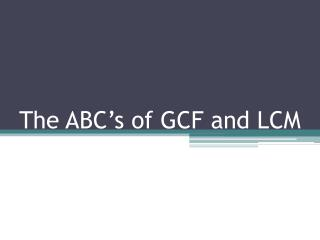DownloadDownload PresentationThe ABC’s of GCF and LCM

# The ABC’s of GCF and LCM

Download Presentation## The ABC’s of GCF and LCM

- - - - - - - - - - - - - - - - - - - - - - - - - - - E N D - - - - - - - - - - - - - - - - - - - - - - - - - - -
##### Presentation Transcript

1. The ABC’s of GCF and LCM

2. GCF = Greatest Common Factor • If the word factor is in the name, I know this has to do with multiplication. • Greatest obviously means the factor with the largest value • Common must mean more than one number has this single factor

3. Greatest Common Factor • The Greatest Common Factor is the largest factor two or more numbers share • How do you find the GCF of 12 and 18? • Using the rules of divisibility, find all of the factors of 12 and 18 • 12= 1, 2, 3, 4, 6, 12 • 18 = 1, 2, 3, 6, 9, 18

4. Greatest Common Factor 12= 1, 2, 3, 4, 6, 12 18 = 1, 2, 3, 6, 9, 18 • Find the numbers that occur in both lists • Choose the number that is the largest • The Greatest Common Factor of 12 and 18 is 6

5. Greatest Common Factor • Find the GCF between 75 and 30 • Use the rules of divisibility to help you 75 –ends in 5 5 * 15 - digits add to 12 (which is divisible by 3) 3 * 25 - follows the identity property 1* 75 The factors of 75 are 1, 3, 5, 15, 25, 75

6. Greatest Common Factor • Find the GCF between 75 and 30 • Use the rules of divisibility to help you 30 –ends in 5 and 0 5 * 6 3 * 10 - is an even number 2 * 15 - follows the identity property 1* 30 The factors of 30 are 1, 2, 3, 5, 6, 10, 15, 30

7. Greatest Common Factor • Compare the two strings of factors and find the largest common factor: 75 = 1, 3, 5, 15, 25, 75 30 = 1, 2, 3, 5, 6, 10, 15, 30 The Greatest Common Factor is 15

8. Greatest Common Factor and Factor Trees • Create factor trees for 18 and 30-remember to divide down to the prime factorization of each number 18 30 3 * 6 5 * 6 3 * 2 3*2 3*3*2 5 * 3 * 2

9. Greatest Common Factor and Factor Trees 18 30 3 * 6 5 * 6 3 * 2 3*2 3*3*2 5 * 3 * 2 • 3 and 2 are common in both prime factorization, therefore 3*2 or 6 is the GCF

10. Practice Time • Math Journal 2 page3 405-407 • Math Practice Book 13.2

11. Least Common Multiple • If the word multiple is in the name, I know this has to do with multiplication • A multiple is the product of two numbers that are multiplied together • 7*3 = 21 21 is the multiple of 7 and 3 • Least means I am looking for the smallest multiple • Common means the multiple must be included in both numbers multiple strings

12. Least Common Multiple • Find the Least Common Multiple of 4 and 6 • List the multiples in order for each number: 4, 8, 12, 16, 20, 24, 28, 32, 36, 40, 44, 48… 6, 12, 18, 24, 30, 36, 42, 48, 54, 60, 66, 72… What is the smallest number that sits in both strings?

13. Least Common Multiple • Find the Least Common Multiple of 4 and 6 • List the multiples in order for each number: 4, 8, 12, 16, 20, 24, 28, 32, 36, 40, 44, 48… 6, 12, 18, 24, 30, 36, 42, 48, 54, 60, 66, 72… What is the smallest number that sits in both strings?

14. Least Common Multiple • Find the LCM of: 6 and 8 3 and 8 6 and 9 24 24 18

15. Practice LCM • Math Practice Book 13.3

16. Using Factor Trees to find the LCM • Use factor trees to find the prime factorization for 18 and 30 18 30 3 * 6 5 * 6 3 * 2 3*2 3*3*2 5 * 3 * 2 • 3*2 repeats in both factor strings

17. Using Factor Trees to find the LCM 3*3*2 3 * 2 * 5 • 3*2 repeats in both factor strings • Eliminate one set of 3 * 2 and multiply the rest of the prime factors together 3 * 3 * 2 * 5 90 The LCM of 18 and 30 is 90

18. Greatest Common Factor and Factor Trees 18 30 3 * 6 5 * 6 3 * 2 3*2 3*3*2 5 * 3 * 2 • 3 and 2 are common in both prime factorization, therefore 3*2 or 6 is the GCF

19. Factoring out the LCM • Find the LCM using factor tree method for: 12 and 42 32 and 20 16 and 24 84 160 48

20. Factoring out the LCM • Practice this method • Math Journal 2 page 408